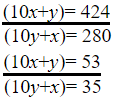# Number System Questions For SSC Mains 2021

Validity: 9 Months
What you will get
Course Highlights
• Based on latest Pattern
• English Medium eBooks
Click to Bookmark
SSC

## Number System Questions For SSC Mains 2021

Here we provide Top-Ten Number System questions and solutions asked by TCS in 2019. Each question is in Hindi/ English.

### Number System Questions For SSC upcoming CGL Tier 1 & Tier 2 Exam

Q1. When a two-digit number is multiplied by the sum of its digits, the product is 424. When the number obtained by interchanging its digits is multiplied by the sum of the digits, the result is 280. The sum of the digits of the given number is :

दो अंकों की एक संख्या को जब इसके अंकों के योग से गुना किया जाता है, तो गुणनफल 424 आता है | जब इसके अंकों को पलटने से बनी संख्या को अंकों के जोड़ से गुना किया जाता  है, तो परिणाम 280 आता है | दी गयी संख्या के अंकों का योग है -

SSC CGL Tier-II 12 September 2019

(a) 6

(b) 9

(c) 8

(d) 7

Q2. If x is the remainder when 361284is divided by 5 and y is the remainder when 496 is divided by 6, then what is the value of (2x-y) ?

यदि x, 361284 को 5 से विभाजित करने पर आने वाला शेषफल है तथा y, 496 को 6 से विभाजित करने पर आने वाला शेषफल है, तो (2x-y) का मान क्या है ?

SSC CGL Tier-II 13 September 2019

(a) -4

(b) 4

(c) -2

(d) 2

Q3. In finding the HCF of two numbers by division method, the last divisor is 17 and the quotients are 1, 11, and 2, respectively. What is the sum of the two numbers?

विभाजन विधि से दो संख्याओं का HCF निकालने के दौरान अंतिम भाजक 17 है तथा शेषफल क्रमशः 1, 11 तथा 2 हैं | दोनों संख्याओं का योग क्या है?

SSC CGL Tier II- 13 September 2019

(a) 833

(b) 867

(c) 816

(d) 901

Q4. If a 10-digit number 5432y1749x is divisible by 72, then what is the value of (5x-4y)?

यदि 10 अंकों की एक संख्या 5432y1749x,  72 से विभाजित है, तो  (5x-4y) का मान क्या है ?

SSC CGL Tier II- 13 September 2019

(a) 14

(b) 15

(c) 10

(d) 9

Q5. What is the remainder when (12797+9797) is divided by 32 ?

जब  (12797+9797) को 32 से भाग दिया जाता है, तो शेषफल क्या आएगा ?

SSC CGL Tier-II 13 September 2019

(a) 4

(b) 2

(c) 7

(d) 0

Q6. Two positive numbers differ by 2001. When the larger number is divided by the smaller number, the quotient is 9 and the remainder is 41. The sum of the digits of the larger number is :

दो धनात्मक संख्याओं में 2001 का अंतर है | जब बड़ी संख्या को छोटी संख्या से भाग दिया जाता  है, तो भागफल 9 आता है तथा शेषफल 41 आता है | बड़ी संख्या के अंकों का योग है :

SSC CGL Tier-II- 13 September 2019

(a) 15

(b) 11

(c) 10

(d) 14

Here are the solutions to the above questions in short methods. it will help you to save your precious time in the exam.

Sol 1. (c)

Let the number = 10x+y

According to the question

(10x+y)(x+y)=424       …(1)

And

(10y+x)(x+y)=280        ……..(2)

Divide equation (1) by (2)350x+35y = 530y + 53x

297x=495y

x/y=5/3

Put this value in any of the equations

(10x+y)(x+y)=[{10(5)+3}(5+3)] = 424

Or

(10y+x)(x+y)=[{10(3)+5}(5+3)] = 280

Clearly 5 and 3 are the desired values and the sum of the digits = 5+3 = 8

Sol 2. (c)

361284= (34)15321

Now, 34= 81. 81 divided by 5 gives remainder 1.

(1)15321=1

So, remainder when 361284 is divided by 5= x = 1

When 4 raised to any power is divided by 6 it will give the remainder 4.

y=4

(2x-y)=2(1)-4= -2

### Sol 3.

Ans. (c)

Since the last divisor is 17 and the quotient is 2, we have the dividend = 17x2 = 34.(Because the last divisor will not leave any remainder)

Now, 34 will become the divisor and 11 will become the quotient, and 17 will be the remainder. Therefore the dividend will be 34 x 11 + 17 = 391

Now, 391 will become the divisor and 1 will be the quotient, and 34 will be the remainder. Therefore the dividend will be 391 x 1 + 34 = 425

So, the numbers are 391 and 425

Required sum = 391+425 = 816

### Sol 4.

Ans. (a)

Since 5432y1749x is divisible by 72 it must be divisible by 9 and 8 (coprime factors of 72) and x must be an even number. So the sum of digits of this number must be divisible by 9 and the last three digits by 8. 5+4+3+2+y+1+7+4+9+x = 35+x+y

x+y must be 1 or 10 as after 35 nearest multiples of 9 are 36 and 45.

Pairs for 1 = (1,0)

x can’t be an odd number. Condition not satisfied.

Pairs for 10 = (1,9), (2,8), (3,7), (4,6), (5,5), (6,4), (7,3), (8,2), (9,1)

Only (6,4) satisfies the given conditions.

⇒ (5x-4y) = 5(6)-4(4) =14

### Sol 15. (d)

(12797+9797) ⇒ (128-1)97+(96+1)97

Remainder from (128-1)97 = (-1)97=-1

Remainder from (96+1)97 = (1)97=1

Final remainder = -1+1 = 0

Sol 6.

Ans. (d)

Let the numbers are x and y.

According to the question

x-y = 2001         ……(1)

9y+41 = x          ………(2)

Put the value of x in eq (1)

9y+41-y=2001

8y = 1960

y=245

x = 9(245)+41 = 2246

Required sum = 2+2+4+6 = 14